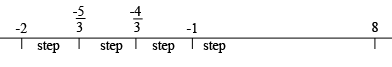SEARCH HOMEMath Central Quandaries & QueriesQuestion from Lauren, a student: Hi there Which term in the sequence -2; -5/3; -4/3 ; -1; ... has a value of 8. Since term 2 and 3 of this sequence contain fractions which can be converted to recurring decimals. What is the best way to work out the common difference here. I do however understand that to work out the nth term of an arithmetic series, the following formula Tn = a + (n-1)d. In this series a = -2Hi Lauren,

I see this sequence as points on the line.The steps are of length

$\frac{-5}{3} - (-2) = \frac{-5}{3} + \frac{6}{3} = \frac{1}{3}$

and

$\frac{-4}{3} - \frac{-5}{3} = \frac{-4}{3} + \frac{5}{3} = \frac{1}{3}$

and so on. Each step is of length $\large \frac{1}{3}.$

How many steps does it take you to get to 8?

$-2 + \mbox{ length of a step } \times \mbox{ number of steps } = 8$

or

$\frac{1}{3} \times \mbox{ number of steps } = 10.$

Thus the number of steps is 30. At this point you need to be careful. You start at -2 and the first step gets you to the second term, the second step gets you to the third term and so on. Thus the $30^{th}$ step get yo to the $31^{st}$ term.

You can use your formula if you prefer it is just that I don't remember formulas well.

PennyMath Central is supported by the University of Regina and the Imperial Oil Foundation.